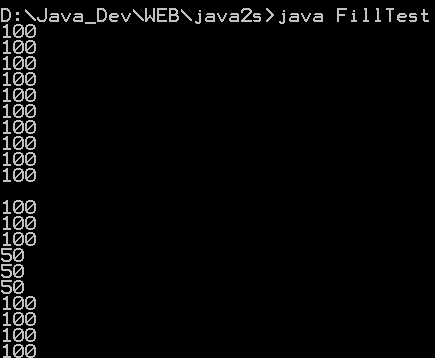# This program demonstrates the various 2D shapes : Shape « 2D Graphics GUI « Java

This program demonstrates the various 2D shapes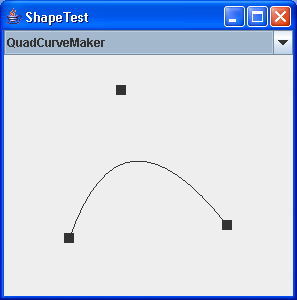```

/*
This program is a part of the companion code for Core Java 8th ed.
(http://horstmann.com/corejava)

This program is free software: you can redistribute it and/or modify
the Free Software Foundation, either version 3 of the License, or
(at your option) any later version.

This program is distributed in the hope that it will be useful,
but WITHOUT ANY WARRANTY; without even the implied warranty of
MERCHANTABILITY or FITNESS FOR A PARTICULAR PURPOSE.  See the
GNU General Public License for more details.

You should have received a copy of the GNU General Public License
along with this program.  If not, see <http://www.gnu.org/licenses/>.
*/

import java.awt.BorderLayout;
import java.awt.EventQueue;
import java.awt.Graphics;
import java.awt.Graphics2D;
import java.awt.Point;
import java.awt.Shape;
import java.awt.event.ActionEvent;
import java.awt.event.ActionListener;
import java.awt.event.MouseEvent;
import java.awt.geom.Arc2D;
import java.awt.geom.CubicCurve2D;
import java.awt.geom.Ellipse2D;
import java.awt.geom.GeneralPath;
import java.awt.geom.Line2D;
import java.awt.geom.Point2D;
import java.awt.geom.Rectangle2D;
import java.awt.geom.RoundRectangle2D;
import java.util.Random;

import javax.swing.JComboBox;
import javax.swing.JComponent;
import javax.swing.JFrame;

/**
* This program demonstrates the various 2D shapes.
* @version 1.02 2007-08-16
* @author Cay Horstmann
*/
public class ShapeTest
{
public static void main(String[] args)
{
EventQueue.invokeLater(new Runnable()
{
public void run()
{
JFrame frame = new ShapeTestFrame();
frame.setDefaultCloseOperation(JFrame.EXIT_ON_CLOSE);
frame.setVisible(true);
}
});
}
}

/**
* This frame contains a combo box to select a shape and a component to draw it.
*/
class ShapeTestFrame extends JFrame
{
public ShapeTestFrame()
{
setTitle("ShapeTest");
setSize(DEFAULT_WIDTH, DEFAULT_HEIGHT);

final ShapeComponent comp = new ShapeComponent();
final JComboBox comboBox = new JComboBox();
{
public void actionPerformed(ActionEvent event)
{
ShapeMaker shapeMaker = (ShapeMaker) comboBox.getSelectedItem();
comp.setShapeMaker(shapeMaker);
}
});
comp.setShapeMaker((ShapeMaker) comboBox.getItemAt(0));
}

private static final int DEFAULT_WIDTH = 300;
private static final int DEFAULT_HEIGHT = 300;
}

/**
* This component draws a shape and allows the user to move the points that define it.
*/
class ShapeComponent extends JComponent
{
public ShapeComponent()
{
{
public void mousePressed(MouseEvent event)
{
Point p = event.getPoint();
for (int i = 0; i < points.length; i++)
{
double x = points[i].getX() - SIZE / 2;
double y = points[i].getY() - SIZE / 2;
Rectangle2D r = new Rectangle2D.Double(x, y, SIZE, SIZE);
if (r.contains(p))
{
current = i;
return;
}
}
}

public void mouseReleased(MouseEvent event)
{
current = -1;
}
});
{
public void mouseDragged(MouseEvent event)
{
if (current == -1) return;
points[current] = event.getPoint();
repaint();
}
});
current = -1;
}

/**
* Set a shape maker and initialize it with a random point set.
* @param aShapeMaker a shape maker that defines a shape from a point set
*/
public void setShapeMaker(ShapeMaker aShapeMaker)
{
shapeMaker = aShapeMaker;
int n = shapeMaker.getPointCount();
points = new Point2D[n];
for (int i = 0; i < n; i++)
{
double x = generator.nextDouble() * getWidth();
double y = generator.nextDouble() * getHeight();
points[i] = new Point2D.Double(x, y);
}
repaint();
}

public void paintComponent(Graphics g)
{
if (points == null) return;
Graphics2D g2 = (Graphics2D) g;
for (int i = 0; i < points.length; i++)
{
double x = points[i].getX() - SIZE / 2;
double y = points[i].getY() - SIZE / 2;
g2.fill(new Rectangle2D.Double(x, y, SIZE, SIZE));
}

g2.draw(shapeMaker.makeShape(points));
}

private Point2D[] points;
private static Random generator = new Random();
private static int SIZE = 10;
private int current;
private ShapeMaker shapeMaker;
}

/**
* A shape maker can make a shape from a point set. Concrete subclasses must return a shape in the
* makeShape method.
*/
abstract class ShapeMaker
{
/**
* Constructs a shape maker.
* @param aPointCount the number of points needed to define this shape.
*/
public ShapeMaker(int aPointCount)
{
pointCount = aPointCount;
}

/**
* Gets the number of points needed to define this shape.
* @return the point count
*/
public int getPointCount()
{
return pointCount;
}

/**
* Makes a shape out of the given point set.
* @param p the points that define the shape
* @return the shape defined by the points
*/
public abstract Shape makeShape(Point2D[] p);

public String toString()
{
return getClass().getName();
}

private int pointCount;
}

/**
* Makes a line that joins two given points.
*/
class LineMaker extends ShapeMaker
{
public LineMaker()
{
super(2);
}

public Shape makeShape(Point2D[] p)
{
return new Line2D.Double(p, p);
}
}

/**
* Makes a rectangle that joins two given corner points.
*/
class RectangleMaker extends ShapeMaker
{
public RectangleMaker()
{
super(2);
}

public Shape makeShape(Point2D[] p)
{
Rectangle2D s = new Rectangle2D.Double();
s.setFrameFromDiagonal(p, p);
return s;
}
}

/**
* Makes a round rectangle that joins two given corner points.
*/
class RoundRectangleMaker extends ShapeMaker
{
public RoundRectangleMaker()
{
super(2);
}

public Shape makeShape(Point2D[] p)
{
RoundRectangle2D s = new RoundRectangle2D.Double(0, 0, 0, 0, 20, 20);
s.setFrameFromDiagonal(p, p);
return s;
}
}

/**
* Makes an ellipse contained in a bounding box with two given corner points.
*/
class EllipseMaker extends ShapeMaker
{
public EllipseMaker()
{
super(2);
}

public Shape makeShape(Point2D[] p)
{
Ellipse2D s = new Ellipse2D.Double();
s.setFrameFromDiagonal(p, p);
return s;
}
}

/**
* Makes an arc contained in a bounding box with two given corner points, and with starting and
* ending angles given by lines emanating from the center of the bounding box and ending in two
* given points. To show the correctness of the angle computation, the returned shape contains the
* arc, the bounding box, and the lines.
*/
class ArcMaker extends ShapeMaker
{
public ArcMaker()
{
super(4);
}

public Shape makeShape(Point2D[] p)
{
double centerX = (p.getX() + p.getX()) / 2;
double centerY = (p.getY() + p.getY()) / 2;
double width = Math.abs(p.getX() - p.getX());
double height = Math.abs(p.getY() - p.getY());

double skewedStartAngle = Math.toDegrees(Math.atan2(-(p.getY() - centerY) * width, (p
.getX() - centerX)
* height));
double skewedEndAngle = Math.toDegrees(Math.atan2(-(p.getY() - centerY) * width, (p
.getX() - centerX)
* height));
double skewedAngleDifference = skewedEndAngle - skewedStartAngle;
if (skewedStartAngle < 0) skewedStartAngle += 360;
if (skewedAngleDifference < 0) skewedAngleDifference += 360;

Arc2D s = new Arc2D.Double(0, 0, 0, 0, skewedStartAngle, skewedAngleDifference, Arc2D.OPEN);
s.setFrameFromDiagonal(p, p);

GeneralPath g = new GeneralPath();
g.append(s, false);
Rectangle2D r = new Rectangle2D.Double();
r.setFrameFromDiagonal(p, p);
g.append(r, false);
Point2D center = new Point2D.Double(centerX, centerY);
g.append(new Line2D.Double(center, p), false);
g.append(new Line2D.Double(center, p), false);
return g;
}
}

/**
* Makes a polygon defined by six corner points.
*/
class PolygonMaker extends ShapeMaker
{
public PolygonMaker()
{
super(6);
}

public Shape makeShape(Point2D[] p)
{
GeneralPath s = new GeneralPath();
s.moveTo((float) p.getX(), (float) p.getY());
for (int i = 1; i < p.length; i++)
s.lineTo((float) p[i].getX(), (float) p[i].getY());
s.closePath();
return s;
}
}

/**
* Makes a quad curve defined by two end points and a control point.
*/
{
{
super(3);
}

public Shape makeShape(Point2D[] p)
{
return new QuadCurve2D.Double(p.getX(), p.getY(), p.getX(), p.getY(), p
.getX(), p.getY());
}
}

/**
* Makes a cubic curve defined by two end points and two control points.
*/
class CubicCurveMaker extends ShapeMaker
{
public CubicCurveMaker()
{
super(4);
}

public Shape makeShape(Point2D[] p)
{
return new CubicCurve2D.Double(p.getX(), p.getY(), p.getX(), p.getY(), p
.getX(), p.getY(), p.getX(), p.getY());
}
}

```

### Related examples in the same category

 1 Creating Basic Shapes 2 fillRect (int, int, int, int) method draws a solid rectangle 3 Creating a Shape Using Lines and Curves 4 Combining Shapes 5 Draw rectangles, use the drawRect() method. To fill rectangles, use the fillRect() method 6 Draw line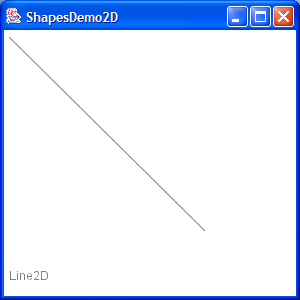7 Draw a Polygon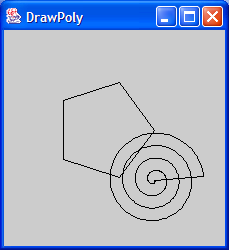8 Draw an oval outline 9 Draw a (Round)rectangle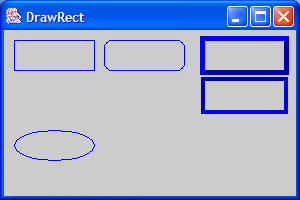10 Fill a polygon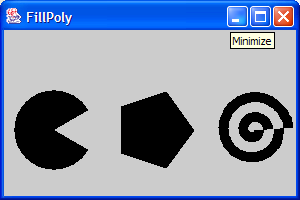11 Fill a solid oval 12 Fill a (Round)rectangle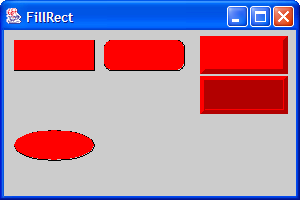13 Change font14 Draw rectangle 2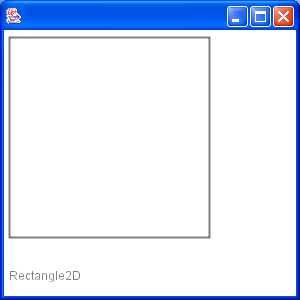15 Draw Arc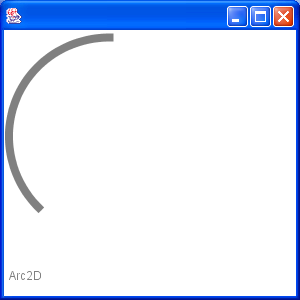16 Draw Ellipse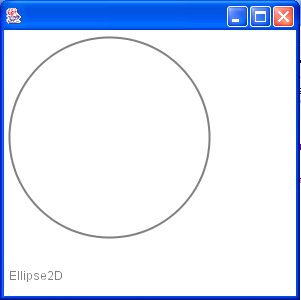17 Fill a Rectangle 2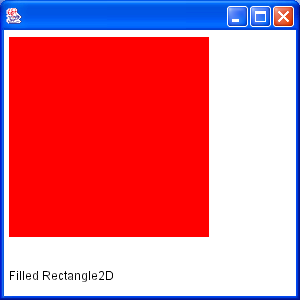18 Fill Arc 2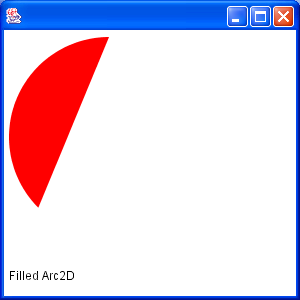19 Draw text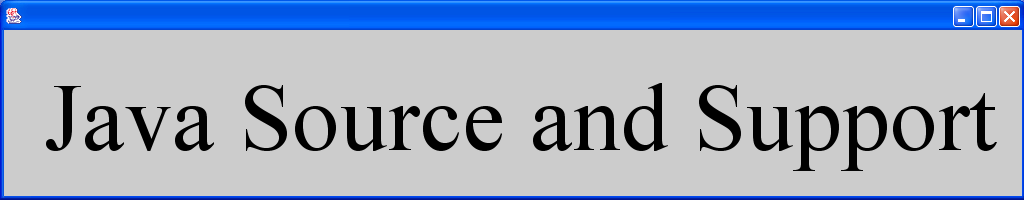20 Draw unicode string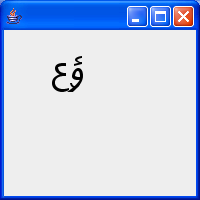21 Shape combine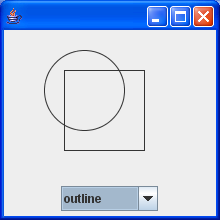22 Effects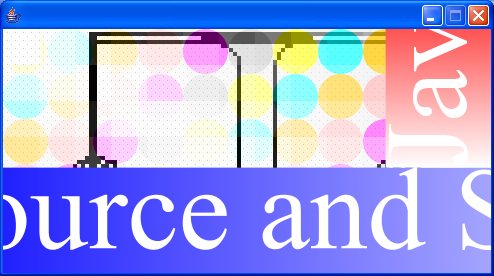23 Mouse drag and drop to draw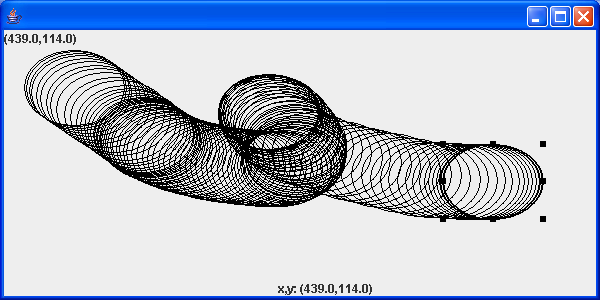24 Arc demonstration: scale, move, rotate, sheer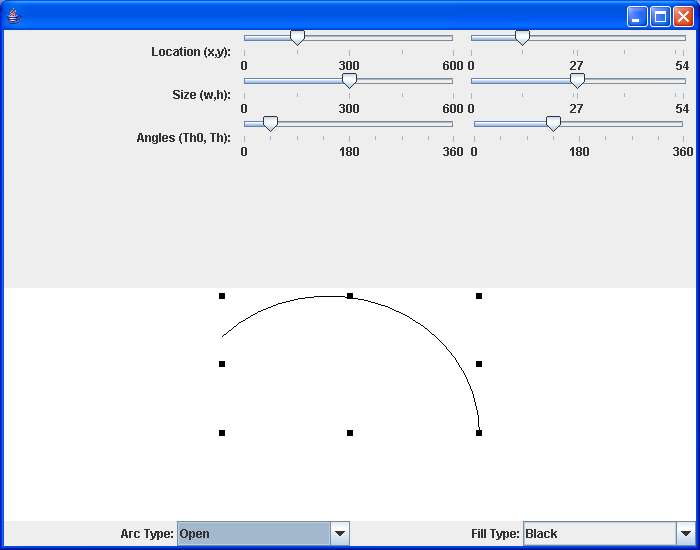25 Hypnosis Spiral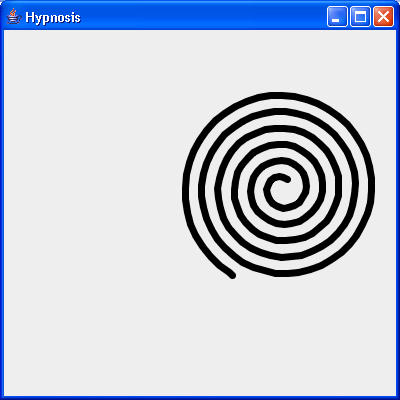26 GlyphVector.getNumGlyphs() 27 Resize a shape 28 Rectangle with rounded corners drawn using Java 2D Graphics API 29 Compares two ellipses and returns true if they are equal or both null. 30 Compares two lines are returns true if they are equal or both null. 31 Creates a diagonal cross shape. 32 Creates a diamond shape. 33 Creates a new Stroke-Object for the given type and with. 34 Creates a region surrounding a line segment by 'widening' the line segment. 35 Creates a triangle shape that points downwards. 36 Creates a triangle shape that points upwards. 37 Generate Polygon 38 Polygon with float coordinates. 39 Polyline 2D 40 Serialises a Shape object. 41 Tests two polygons for equality. If both are null this method returns true. 42 Union two rectangles 43 Calculate Intersection Clip 44 Draws a shape with the specified rotation about (x, y). 45 Checks, whether the given rectangle1 fully contains rectangle 2 (even if rectangle 2 has a height or width of zero!). 46 Reads a Point2D object that has been serialised by the writePoint2D(Point2D, ObjectOutputStream)} method. 47 Returns a point based on (x, y) but constrained to be within the bounds of a given rectangle. 48 RectListManager is a class to manage a list of rectangular regions. 49 Fill Rectangle2D.Double and Ellipse2D.Double